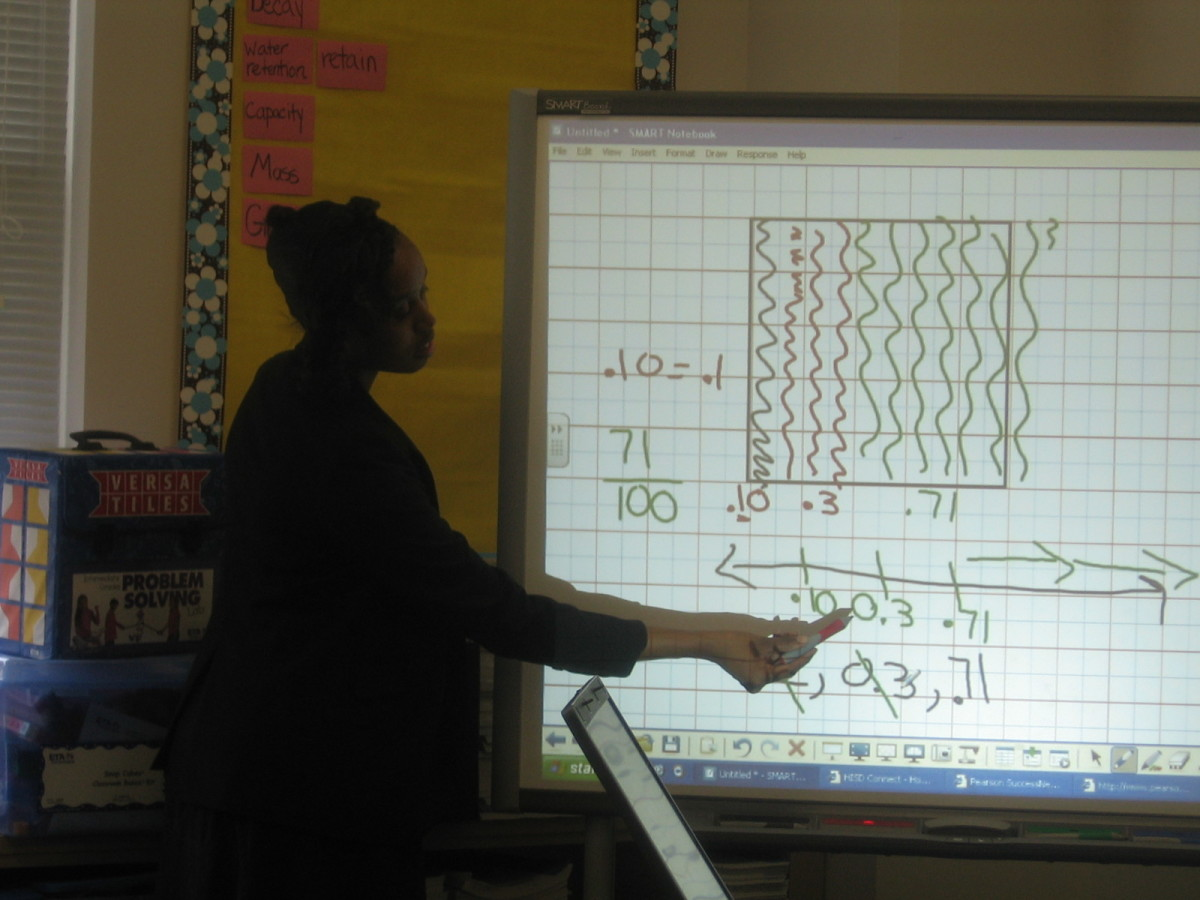Updated date:

# Similarities between fractions and decimalsHere using a Smartboard in the classroom I show students how making a 10X10 grid can help with understanding fractions.

## Why are Fractions & Decimals the Same?

Often students struggle to see the similarities between fractions and decimals. Both terms describe parts of a whole. Decimals are a based ten system, being so using money to teach decimals makes it very easy to explain. Use graph paper to make a 10 X 10 grid. Use this as a visual to see the similarities. For example, graph 75/100 which is the same thing as .75(seventy-five hundredths), or using money you can say 75 pennies out of 100. Use the place value chart to also show how the value of numbers vary depending on their location. A place value chart is a chart that shows values to left and right of the decimal point. Numbers before the decimal are whole numbers and numbers after the point are fractions. In the chart below the number two hundred thirty one million, thirteen thousand, four hundred thirty two and 13 hundredths is displayed with each digit in its proper place value. The decimal point separates the whole numbers from the decimals or fractions. How is one tenth a fraction? Well we say the fraction when we say the name of a decimal. If you say .68 that is the fraction 68 out of 100. Thinking of money that is 68 pennies out of 100. If you shade in 68 units on a 10X10 grid you can visually see a selected portion of a whole.

## Place Value Chart

Millions PeriodThousands PeriodOnes PeriodDecimal Point (.)TenthHundredthThousandth

hundred,ten,one

hundred,ten,one

hundred,ten,one

231,

013,

432

.

1

3

## How to Convert Fractions into Decimals?

No matter what the fraction is it can always be converted into a decimal. This can be done using the division operation. The numerator is the dividend, and the denominator is the divisor. When converting proper fractions with smaller numerators and larger denominators, the answer is always a decimal. For example if I have the fraction 2/5, I would divide 2 by 5. This would equal .40. In the table below frequently used fractions are shown with their decimal and percentage equivalences. I also included an every day thing that might help students relate better, pizza. For an activity you can have students create a similar chart, or you can give students a chart with missing information for them to figure out and complete. Where it says pizza they can draw what the pizza would look like for each fraction.

## Equivalent Table for Frequently Used Fractions

 Fraction slices of pizza Decimal Percent Division 1/10 1 of 10 .10 10% 1 divided 10 1/3 1 of 3 .33 33% 1 divided by 3 1/4 1 of 4 .25 25% 1 divided by 4 1/2 1 of 2 .50 50% 1 diviided by 2 3/4 3 of 4 .75 75% 3 divided by 4 1/1 1 of 1 1.00 100% 1 divided by 1